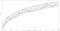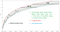# Summary

“The price model presented here estimates bitcoin to reach 100k\$ in August 2026, with a range of uncertainty of nine years: not earlier than January 2021 and no later than February 2030.”

“The model also estimates bitcoin to reach 1M\$ in November 2036, with an uncertainty of fifteen years: not earlier than November 2027 and no later than July 2042.”

“Any outcome in these ranges is VERY POSITIVE, the most conservative outcome still returning an annualized profit of 23% every year over a 23 year period.”

# Introduction

“How fast will bitcoin grow from here?” must be the foremost question of most bitcoin investors. Every day you can find people trying to answer this question, but bitcoin’s high volatility and fractal hype cycles make these point price predictions near useless. But by embracing the uncertainty in bitcoin’s price development, standard regression methods can deliver range price estimates that will stand a far better chance of becoming reality.

In this article [a] I start with the work of fellow bitcoin researchers having used power law models, [b] make a simple but effective change to the power law model and [c] apply that new model to deliver a variety of range estimates (dates when milestone prices are reached, and cumulative annual growth rates). You might find the outcomes informative and useful in your investment decisions.

# Methods

## Price model

Bitcoin has an approximate price history of almost 10 years now: 3319 daily data points in my data source, more than sufficient to apply some basic mathematical modeling. There are plenty of price models to choose from. I mention two here:

1. PlanB’s excellent valuation model based on scarcity (stock to flow ratio)
2. DaveTheWave’s price models based on two-parameter logarithmic regression

Although the models of these two authors are intrinsically different, both use the equation:

or in its power form:

to an array of input data (x, y). A and B are constants in these equations defining a linear function between log(x) and log(y) and are determined by minimizing the least summed squared distances between model and realized observations. Linear dependency between the logarithms of two entities is a property of a power law relationship. Power law relationships in price models for bitcoin make absolute sense fundamentally, as PlanB clearly explains and shows based on the incremental scarcity of bitcoin.

## Adding a time shift parameter

Although my initial attempts reconstructed the published charts of the above models quite satisfactory, and I thus could have chosen to continue with these models, I got an improvement in the quality of my fits by adding a third parameter S to the equation:

or in its power form:

Parameter A is the slope of the line between log(x+S) and log(y), B is the intercept of this line (crossing of the Y-axis), and S controls the point of singularity of the input logarithmic function. S shifts the time origin of bitcoin, so that instead of assuming that the first day of the price data matches the time origin, or that the time origin matches the date of the genesis block, we allow the model to determine the time origin of bitcoin by itself.

## Fit method

When using a two-parameter power-law model, it suffices to perform a linear fit between log(price) and log(time). But with the addition of the third parameter a non-linear fit method is needed, which can be done by the Levenberg-Marquardt algorithm, that can fit any parametric non-linear equation. I used the LabVIEW platform to do this, but other platforms can do this just as well.

# Results

## The fit

Per today, the fitted equation applied to y = daily bitcoin price and x = time in days since the earliest time point in bitcoin’s historic price data (17 Jul 2010) to the price of yesterday (1 Sep 2019) is:

or in its power form:

R² (the coefficient of determination) is 0.935, meaning that the smooth curve as defined by the three parameters accounts for 93.5% of the total variance in bitcoin’s price history. Not bad. The green line in the chart below shows this fit, on a lin (time) vs. log (price) scale, and where time is restored to its date form.The Levenberg-Marquardt algorithm was used to fit the three-parameter power-law curve to the daily price of bitcoin (BLACK line) from 17 July 2010 to 28 August 2019 (GREEN curve). The RED curve is 7x the price of the green line, the BLUE curve is 0.4x the price of the green line, to obtain a bandwidth from which range estimates can be derived.

Note that 10^(-13.43 ) is a small number, and(x+312)^4.860 is a time-varying large number. Multiplication of these two does the magic of producing the green line above. Let’s reassure by applying this formula with the date of today, 2 Sep 2019, which is 3334 days after 17 Jul 2010, the start of our bitcoin price data. The formula is:

This is today’s model price. The actual price today is 9941.97 \$ (BRR Bitcoin Reference Rate). So according to the model, bitcoin today is 31% overvalued. Business as usual for bitcoin.

## Date range estimates

With this satisfactory and plausible model to fit bitcoin’s price based on time, I continued by setting a bandwidth around the model price, as wide as needed to also just include the tops (booms) and bottoms (busts) in all of bitcoin’s price history. After trying out several methods for this, it turned out that tops in bitcoin price occurred consistently at approximately 7 times the model bitcoin price (the red line in the chart above), while bottoms occurred consistently at approximately 0.4 times the model bitcoin price (the blue line). By using 7 and 0.4 as multiplication constants, a price-range is obtained every day. In the chart it seems as if this band of ranges expands with increasing time, but that is an optical illusion: each vertical line between the red and blue lines has equal length. Now the band is there, it can easily be back-projected on the time axis (using the inverse non-linear equation) at any given price to produce a time-range. The chart illustrates this method graphically at the 100k\$ and 1M\$ levels, as the whiskers.This is the same chart, but the x-axis is much expanded into the future so that the full price band of bitcoin reaches 1 M\$. The green dots are the model date predictions when bitcoin reaches 100k\$ and 1M\$ and the horizontal whiskers are the range date predictions.

Using this method, the estimated dates and date-ranges for the milestone prices of 100k\$ and 1M\$ are:

• Date to reach 100k\$: 15 Aug 2026 (11 Jan 2021 to 20 Feb 2030)
• Date to reach 1M\$: 23 Nov 2036 (30 Nov 2027 to 20 Jul 2042)

These ranges are much more informative than any point prediction, because these ranges are directly generated by strictly rational methods, based upon extrapolation of all we know about bitcoin price discovery so far, and by using a power-law model that has been shown quite plausible on fundamental and technical grounds by others.

## Not earlier and No later

Because this model estimates the maximum bandwidth of bitcoin’s price at every point in time, it also gives these quite stringent clues:

• Before 11 Jan 2021 bitcoin’s price is not likely to reach 100k\$
• Before 30 Nov 2027 bitcoin’s price is not likely to reach 1M\$
• After 20 Feb 2030 bitcoin’s price is likely never to fall below 100k\$
• After 20 Jul 2042 bitcoin’s price is likely never to fall below 1M\$

## Annual Growth range estimates

The model can also be used to estimate cumulative annual growth rates (CAGRs) on an investment made today in bitcoin while it is underway to these milestones:

• CAGR to reach 100 k\$: 40% (25% to 455%)
• CAGR to reach 1M\$: 31% (23% to 76%)

The results obtained for the 1M\$ milestone tell us that in the least steep growth scenario, bitcoin still delivers an average annual growth of 23% over the course of 22.9 years. The steepest growth scenario gives an average annual growth of 76% over the course of 8.3 years, while the model growth scenario delivers 31% over 17.2 years.

## The time origin of bitcoin and the validity of the model

Parameter S got a value of 312 in the best fit. My historic bitcoin price data started at 17 Jul 2010 (x=0), so S=312 corresponds to 8 Sep 2009 (17 Jul 2010 minus 312 days) as the starting date for bitcoin that the model picked. This is a few months later than the date of bitcoin’s genesis block, which is 3 Jan 2009, used by others in their price models as a constant (quite a natural choice btw). During these first months the number of transactions and the number of addresses were very low, so its unlikely that price discovery had started. The model was free to select any date as its starting date, even dates before the genesis. The fact that it selected a date so close after the genesis, strengthens the hypothesis that bitcoin price data does indeed follow a power-law relation, which is very hard to proof and can’t only be concluded from the linear shape on the log(price)-log(time) scale alone. Phrased alternatively: the fact that the three parameter model picks such proper starting date increases the trust in the model.

# Conclusions

These range estimates are obtained by projecting the complete currently available bitcoin price data into the future via a three-parameter power-law model, setting landmarks to orient on the future price action of bitcoin. A CAGR in dollar value of 23% over 23 years from now on (quite easy to remember ;-) as the most conservative estimate, doesn’t look like a bad investment. Because the model parameters update gradually with every newly realized daily price point of bitcoin, the estimated dates and annual growth rates will slowly fluctuate over time. But if bitcoin keeps doing what it has been doing for almost 10 years, these ranges should keep quite stable. I’m on the lookout for that and plan to publish updates to this article with newly fitted ranges at regular intervals.

[Added 11 Dec 2019 by the author: M. Burger (BurgerCrypto.com) criticized this model openly on Twitter for containing a date origin. When explaining to him that the estimate for this date origin at the moment is 312, corresponding to 8 Sep 2009, he responded by asking if I could not see ‘how crazy that is’. My answer here is that this is not crazy at all. It’s just as crazy as assuming that the slope (parameter a) is 4.86, or the intercept (parameter b) is 13.4. In fact, since the model was free to choose any date as its starting date, the fact that it is ‘only’ a few months off the genesis block date strengthens the validity of the model, and M. Burger’s criticism for ‘hand picking’ a best fitting starting date is not valid. The Stock to Flow model, supported to the max by him, estimates two parameters based on a power law. Let’s see in the future how ‘crazy’ these values turn out to be.]

## Quantodian Publications

A collection of papers by selected contributors

## Quantodian Publications

A collection of papers by selected contributors

Written by

## InTheLoop

Exploring bitcoin data for fun and for profit## Quantodian Publications

A collection of papers by selected contributors